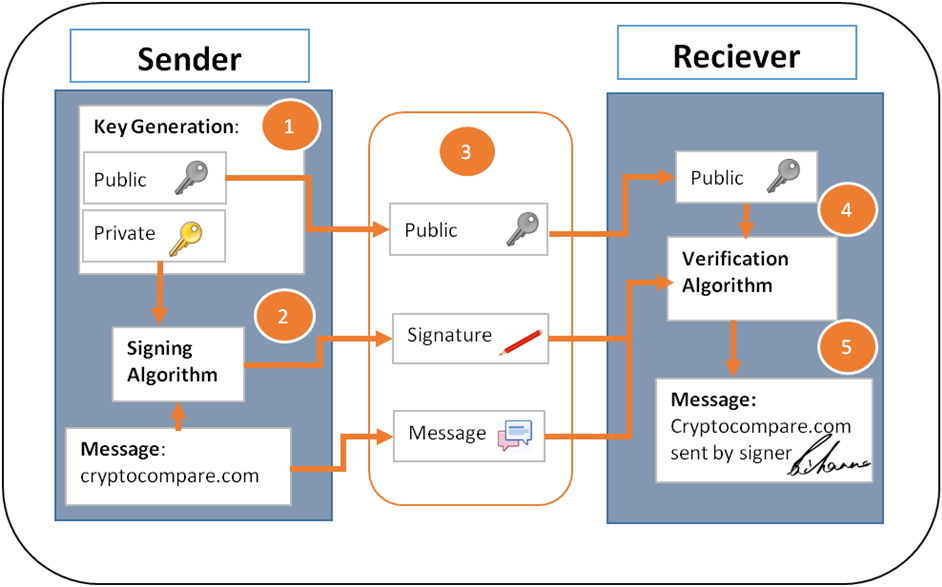# How do digital signatures in Bitcoin work?

12 Feb 2015A Bitcoin digital signature and its verification is one of the main key secrets behind the Bitcoin protocol.

It allows non-repudiation as it means the person who sent the message had to be in possession of the private key and so therefore owns the Bitcoins – anyone on the network can verify the transaction as a result.

But how does it work?

The private key creates the public key, which in turn creates the address – and due to some clever mathematics using elliptic curves and modular arithmetic in finite fields the process is achieved. First though let’s remind ourselves of the process.The sender generates a private key and public key. They then sign the message with the signature and send their public key, the signature and the message to the network (as the network is peer to peer each full node in the network validates each transaction) – The node or receiver then checks using the verification algorithm that the message has been signed by the sender, which can only be done by the holder of the private key to the public key that is sent.

Using elliptic curves and their properties the signer, or sender, creates three points – remembering that the Bitcoin curve is defined as below:

y2=x3+7 mod n where n=1.158x1077

1.1 Take the message and convert it to a number by hashing it – then multiply by the generator point.

Mp (Xm,Ym) = (Message Hash=Mh)* G(Xg,Yg)

1.2 Choose a random number and create a random point by multiplying the generator point.

Rp (Xr,Yr) = (Random Number=Rn)* G(Xg,Yg)

1.3 We take the random point, Xr, just the x coordinate and multiply by the Public key – which remember is a point in its own right derived from the generator point and private key -which is a random number.

Pu (Xk,Yk) = (Xm)* Public Key(Xpub,Ypub)= (Xm)*(Private Key=Pr)* G(Xg,Yg)

After this the signer creates the signature factor using the Bitcoin private key, the random point x coordinate, the message hash, and the random number used to generate the random point.

Signature Factor=Sf=(Mh+Xr*Pr)/Rn mod n

The signature is (Sf, Xr), which along with the public key & message is sent to the network or receiver who then makes some calculations of their own, which are as follows.

2.1Take the message and hash it and divide by the signature factor.

U1 = ((Message Hash = Mh))/Sf

2.2 Calculate the Random number divided by the signature factor.

U2 = ((Xr))/Sf

Then calculate the random point using the following equation – the same point calculated by the signer in step 1.2:

Rp (Xr,Yr) = (U1)* G(Xg,Yg)+ (U2)* Public Key(Xpub,YPub)

If Xr of the sender ≡ Xr of the receiver then the signature is valid and the transaction had to have been sent by the holder of the private key. The equation above can be substituted in the following way to prove this.
Substitute in the following equations:

Signature Factor=Sf=(Mh+Xr*Pr)/Rn mod n
Public Key(Xpub,Ypub)= (Private Key=Pr)* G(Xg,Yg)
U1 = ((Message Hash = Mh))/Sf
U2 = ((Xr))/Sf

Into equation 2.3 to get:

Rp (Xr,Yr) = (((Message Hash = Mh))/Sf)* G(Xg,Yg)+ ((Xr))/Sf* (Pr)* G(Xg,Yg)
Rp (Xr,Yr) =((Mh)+Xr*Pr)*( ( G(Xg,Yg)))/Sf

Rp (Xr,Yr)=( ((Mh)+Xr*Pr))/(((Mh)+Xr*Pr) )*Rn* G(Xg,Yg)=Rn* G(Xg,Yg)

This proves that the signature came from the holder of the private key, and that the transaction is valid. A lot of this relies on wallets not using the same random number (Rn) to be generated for two transactions. If this was the case then the above equations can be solved to find the Private key number.